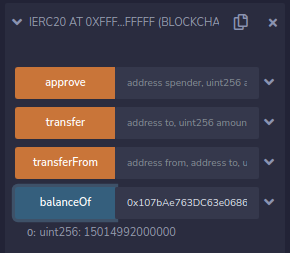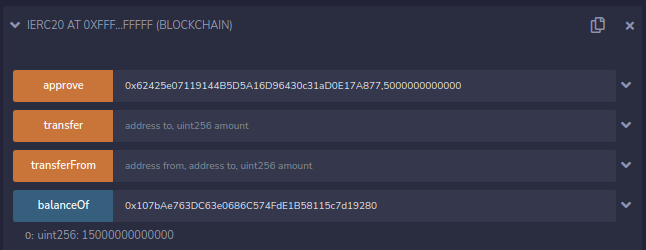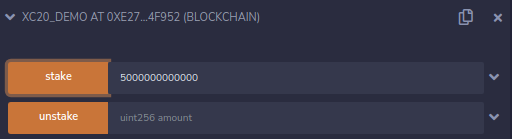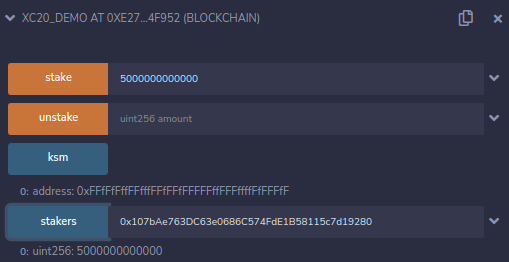# XC20 in Smart Contracts

So far we’ve successfully transferred assets from Kusama to Shiden and shown how we can access those assets the same as any other within MetaMask. Now we will take it one step further and demonstrate how to use these assets with smart contracts.

Here is an example staking contract we’ll be using for the demonstration (for DOT, replace ksm with dot):

note

XC20 address of relay chain token (KSM and DOT) is 0xFFFFFFFFFFFFFFFFFFFFFFFFFFFFFFFFFFFFFFFF (because Id 340,282,366,920,938,463,463,374,607,431,768,211,455 to hex is FFFFFFFFFFFFFFFFFFFFFFFFFFFFFFFF).
for example 0xFFFFFFFFFFFFFFFFFFFFFFFFFFFFFFFFFFFFFFFF will be converted to 0xFFfFfFffFFfffFFfFFfFFFFFffFFFffffFfFFFfF

// SPDX-License-Identifier: MITpragma solidity ^0.8.0;// A partial ERC20 interface, just for the demo purposesinterface IERC20 {    function balanceOf(address owner) external view returns (uint256);    function approve(address spender, uint256 amount) external returns (bool);    function transfer(address to, uint256 amount) external returns (bool);    function transferFrom(address from, address to, uint256 amount) external returns (bool);}contract XC20_Demo {    IERC20 public ksm = IERC20(0xFFfFfFffFFfffFFfFFfFFFFFffFFFffffFfFFFfF);    mapping(address => uint256) public stakers;    constructor() payable {}    function stake(uint256 amount) public {        require(ksm.transferFrom(msg.sender, address(this), amount));        stakers[msg.sender] += amount;    }    function unstake(uint256 amount) public {        require(stakers[msg.sender] >= amount);        require(ksm.transfer(msg.sender, amount));        stakers[msg.sender] -= amount;    }}

Using the partial ERC20 interface (all methods are actually supported), we can check the balance of the account we used previously: 0x107bAe763DC63e0686C574FdE1B58115c7d19280The amount shown is the same amount we see in MetaMask, 15 KSM in total.

Now, Lets stake 5 KSM in our simple staking dApp. The first step will be to approve the staking demo contract 0x62425e07119144B5D5A16D96430c31aD0E17A877 for the transfer, using the IERC20 interface:Now we can stake 5 KSM.After the operation is complete, we can check the staked amount.We’ve successfully staked 5 KSM!

To summarize what we’ve done so far:

1. Transferred KSM tokens from Kusama to Shiden.
2. Verified the tokens are present within MetaMask.
3. Confirmed we are able to manipulate the XC20 tokens in the same way as other ERC20 tokens, via smart contract.